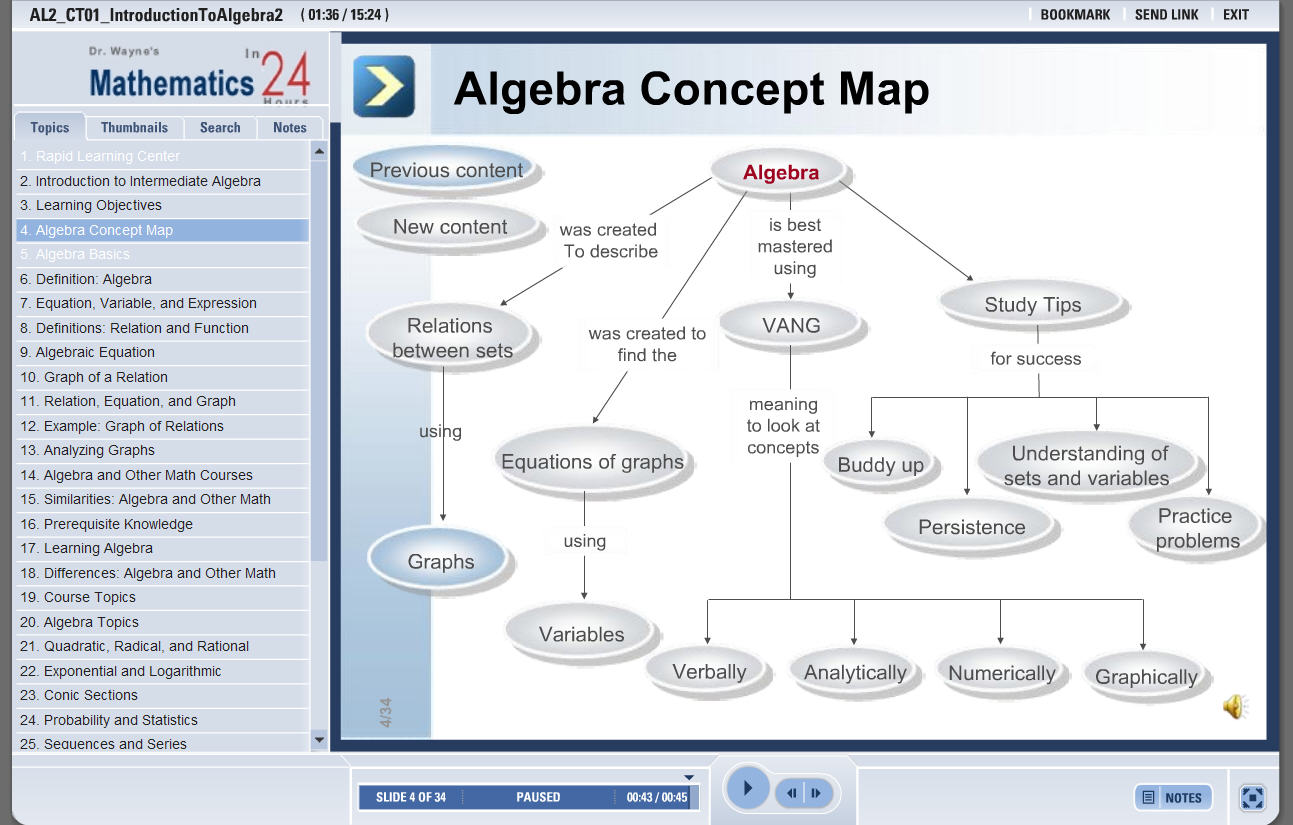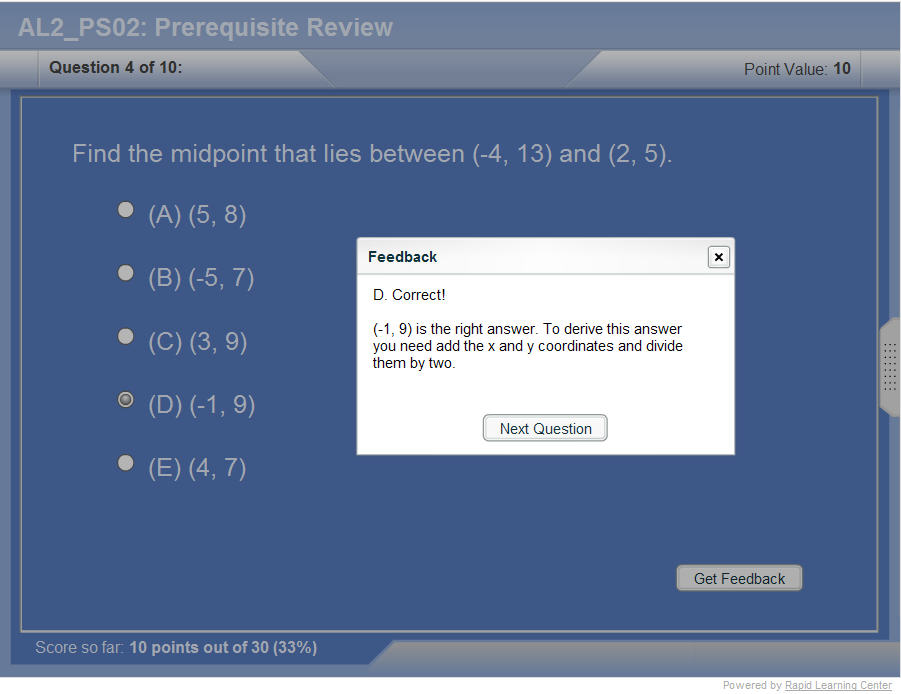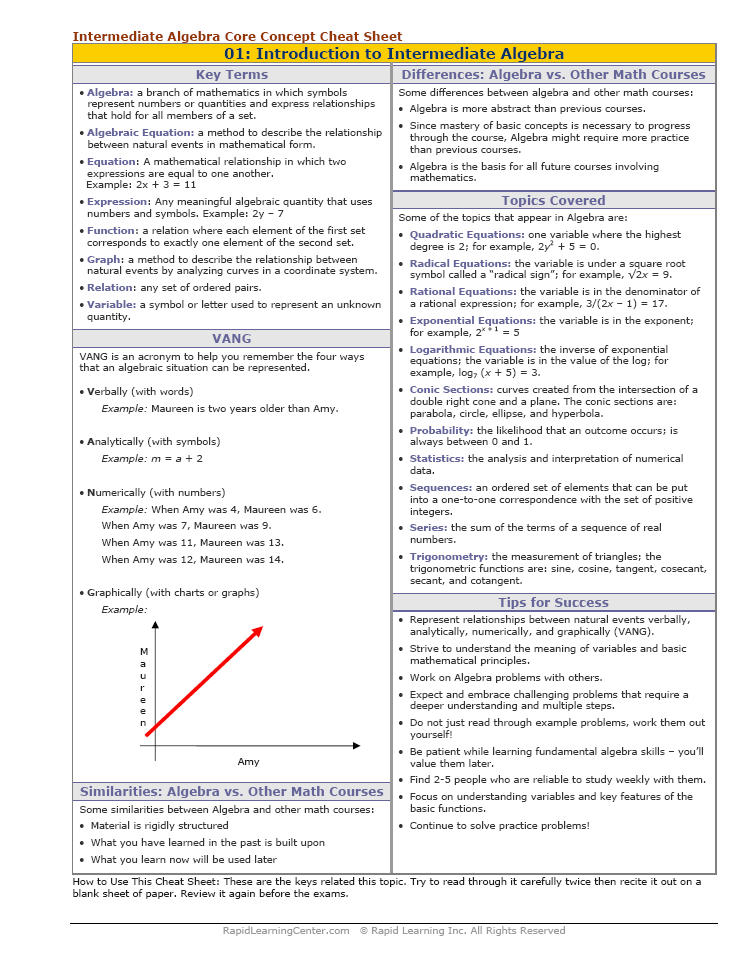How to Learn in 24 Hours?The Rapid Learning Movie

 Need Help? M-F: 9am-5pm(PST): Toll-Free: (877) RAPID-10 US Direct: (714) 692-2900 Int'l: 001-714-692-2900 24/7 Online Technical Support: The Rapid Support Center Secure Online Order:Need Proof? Testimonials by Our Users

 Rapid Learning Courses: MCAT in 24 Hours (2015-16) USMLE in 24 Hours (Boards) Chemistry in 24 Hours Biology in 24 Hours Physics in 24 Hours Mathematics in 24 Hours Psychology in 24 Hours SAT in 24 Hours ACT in 24 Hours AP in 24 Hours CLEP in 24 Hours DAT in 24 Hours (Dental) OAT in 24 Hours (Optometry) PCAT in 24 Hours (Pharmacy) Nursing Entrance Exams Certification in 24 Hours eBook - Survival Kits Audiobooks (MP3)

 Tell-A-Friend: Have friends taking science and math courses too? Tell them about our rapid learning system.Home »  Mathematics »  Intermediate Algebra

Trigonometric Equations

 Topic Review on "Title": Trigonometric equation: Trigonometric is an equation consisting of trigonometric functions.  Solutions of trigonometric equations: Solutions of trigonometric equations are the values of x where the trigonometric equations are defined within the domain of the trigonometric functions.

Rapid Study Kit for "Title":
 Flash Movie Flash Game Flash Card Core Concept Tutorial Problem Solving Drill Review Cheat Sheet"Title" Tutorial Summary : Solving trigonometric equations is discussed in this tutorial with the aid of examples. Using trigonometric identities from this previous tutorial a trigonometric equation can be simplified. The properties of the trigonometric functions are used in the solving process of trigonometric equations. Specific cases of trigonometric equations such as the homogenous equations are shown in the examples and solved with the use of trigonometric identities. Strategies to solve a trigonometric equation use algebra and specified trigonometry principles.

 Tutorial Features: Specific Tutorial Features: • Step by step solving of trigonometric equations. • Typical examples of trigonometric equations. Series Features: • Concept map showing inter-connections of new concepts in this tutorial and those previously introduced. • Definition slides introduce terms as they are needed. • Visual representation of concepts • Animated examples—worked out step by step • A concise summary is given at the conclusion of the tutorial.

 "Title" Topic List: Definition of a trigonometric equationSolving trigonometric equations Type sin(x) = a Type cos(x) = a Type tan(x) = a Type sin(x) = sin(a) Type cos(x) = cos(a) Type tan(x) = tan(a) Type a.cos(x) + b.sin(x) = c

See all 24 lessons in Intermediate Algebra, including concept tutorials, problem drills and cheat sheets:
Teach Yourself Intermediate Algebra Visually in 24 Hours